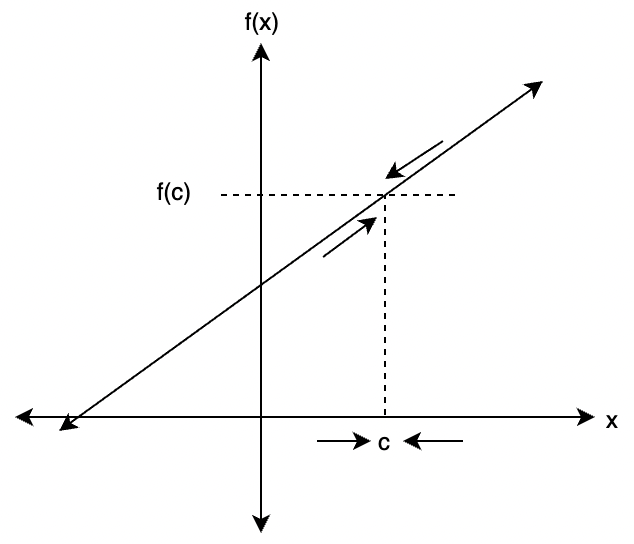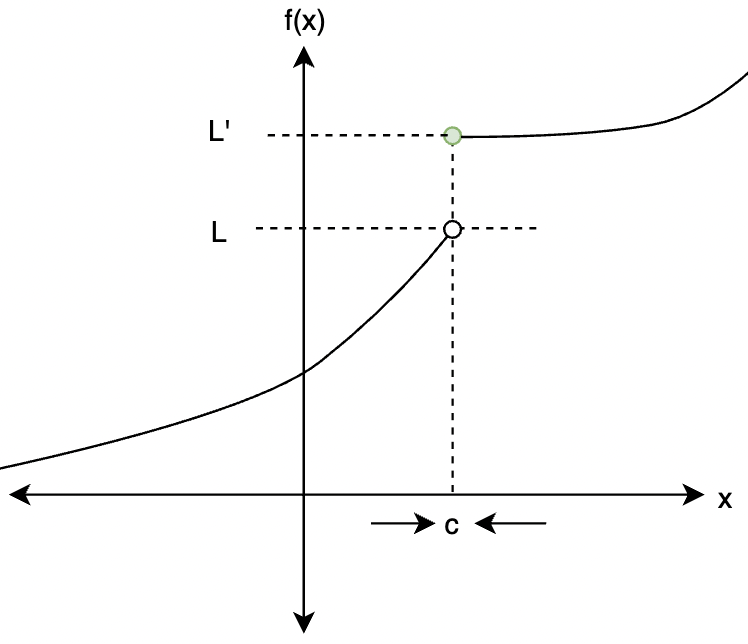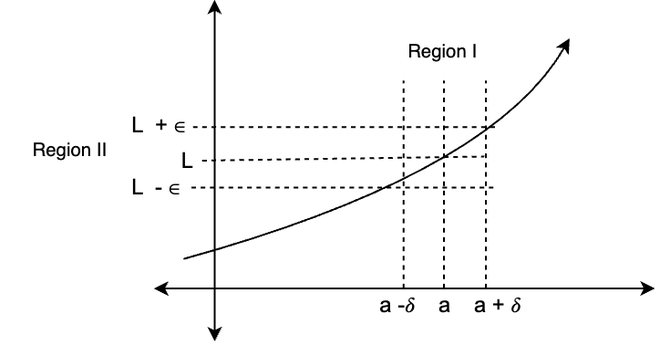GeeksforGeeks App
Open AppBrowser
Continue

# Formal Definition of Limits

Intuitively limit for a function f(x) at a point x, means the value that function seems to be approaching as one moves towards the point x. The understanding of these concepts leads to the development of some fundamental concepts of calculus – continuity, derivatives, and integrals. There are three kinds of limits — Left-hand Limit, Right-Hand Limit, and both. Limits are defined more precisely and in a mathematical way using the epsilon-delta definition. Let’s look at the definition of the limit at the points with finite limit, infinite limit, and limit at infinity.

### Intuition of Limits

The limit of a function f(x) at a particular point x, describes the behavior of that function very close to that particular point. It does not give the value of the function at that particular point, it just gives the value function that seems to be taking on at that point. The figure below presents a straight line and the limit of that function at point x = c, shown graphically.Notice in the figure, as one approaches the point x = c from either left-hand side or right-hand side. The value of the function is changing. As one reaches point c, the value of the function seems to be converging to f(c).

Sometimes functions seem to be taking on some value in the limit and another value on the actual point. This usually happens in the functions which are not continuous and have jumped in their values. For example, consider the function given in the figure below,In this function, while approaching from the Left-Hand side, the function seems to be taking on the value, thus limit evaluates to L, but the actual value of the function at that point is f(c) = L’. Observe that, approaching from the right-hand side, the limit evaluates to f(c) = L’.  This proves that it is not necessary that the value of the function at a particular point is the same as the limit of the function at that particular point.

### Formal Definition of Limits

Let’s consider a function f(x), the function is defined on the interval that contains x = a. The limit of the function at x = a is denoted as,. This is written as “Limit of f(x) when x tends to a”. Notice the words “tends to”, “approaches to” that are usually used to define the limits are not precise, and thus it is impossible to write that mathematically. So, a definition is needed which formalizes the notion for these phrases and words.

Building the Idea

Consider the following function case,The figure shows the values of a function f(x) at three different points which are close to each other.represents the change in the value of x, and the ∈ shows the change in the values the limit of the function at these points. These parameters formalize the notion of the points being really close to each other and the meaning of the phrases like x approaching the point x = a. So, using the formal definition of the limits can be written down.

Definition of Limits

The definition given below is called the “epsilon-delta” definition.

For the function f(x) defined on an interval that contains x =a. Then we say that,If for every number epsilon(∈) which greater than zero, there is some positive number deltasuch that,

| f(x) – L | < ∈ where 0 < |x – a| <What The definition is trying to say can be explained with the figure above. Let’s assume that the limit doesn’t exist. According to the definition, there is a certain number ∈ > 0, that we pick. The two horizontal lines in the figure represent L + ∈ and L – ∈. After that, the definition says, that there is another number> 0 out there that we need to determine. It allows us to add those vertical lines in the figure representing a +, a –. For any point that is there between region I,

0 < |x — a| <If we now identify the point on the graph that our choice of x gives, then this point on the graph will lie in the intersection of the pink and yellow region. Now the value of the function will lie between,Using the definition to calculate the Limits

As an example, let’s consider a function f(x) = x2. Using the limit definition mentioned above, prove thatFor this case, L = 0 and a = 0. Consider any arbitrary number ∈ > 0.

|x2 – 0| < ∈

⇒|x2| < ∈

The goal is to find a number, such that,

| x2 | < ∈ where 0 < |x| <| x2 | < ∈

⇒ |x| < √∈

We can choose our= √∈

For verification, one should check that for the given condition with, the value of the expression should not change more than ∈. In this particular case, it means that we need to make sure that,So, let’s use the previously derived result |x| < √∈. Squaring it,

| x2 | < ∈

This is the result we were looking for. Hence, verified.

Let’s see some sample problems with these concepts.

### Sample Problems

Question 1: For the given function f(x), Findf(x) =Solution:

Using the substitution rule,

f(x) =Question 2: For the given function f(x), Findf(x) =Solution:

Using the substitution rule,

f(x) =⇒1

Question 3: For the given function f(x), Findf(x) =Solution:

Using the substitution rule,

f(x) =⇒1

Question 4: For the given function f(x), Prove using the epsilon delta definition of the limit,f(x) = 5x -4

Solution:

For this case, L = 6 and a = 2. Consider any arbitrary number ∈ > 0.

|5x -4 – 6 | < ∈

⇒|5x -4 – 6| < ∈

The goal is to find a number, such that,

|5x -4 – 6| < ∈ where 0 < |x – 2| <|5x -4 – 6| < ∈

⇒ 5 | x – 2| < ∈

⇒ |x – 2| <We can choose our=For verification, one should check that for the given condition with, the value of the expression should not change more than ∈. In this particular case, it means that we need to make sure that,

|5x -4 – 6 | < ∈

Let’s take the expression and use the derived result.

⇒ 5 | x- 2| < 5⇒|5x -4 – 6 | < ∈

Question 5: For the given function f(x), Prove using the epsilon delta definition of the limit,f(x) = √x

Solution:

For this case, L = 0 and a = 0. Consider any arbitrary number ∈ > 0.

|√x – 0| < ∈

⇒|√x| < ∈

The goal is to find a number, such that,

| √x | < ∈ where 0 < |x| <| √x | < ∈

⇒ |x| < ∈2

We can choose our=∈2

For verification, one should check that for the given condition with, the value of the expression should not change more than ∈. In this particular case, it means that we need to make sure that,

|√x| < ∈

So, let’s use the previously derived result |x| < ∈2. Taking the square root,

| √x | < ∈

Question 6: For the given function f(x), Prove using the epsilon delta definition of the limit,f(x) = ex

Solution:

For this case, L = 1 and a = 0. Consider any arbitrary number ∈ > 0.

|e – 1| < ∈

⇒|ex – 1| < ∈

The goal is to find a number, such that,

|ex – 1| < ∈ where 0 < |x| <|ex – 1| < ∈

⇒ -∈ <ex – 1 < ∈

⇒ 1 -∈ <ex < ∈ + 1

⇒log (1 – ∈) < x < log(∈ + 1)

Here, ∈ < 1 for the left-hand side inequality to be valid.

We can choose our=min{log (1 – ∈), log(∈ + 1)} = log(1 + ∈)

For verification, one should check that for the given condition with, the value of the expression should not change more than ∈. In this particular case, it means that we need to make sure that,

|ex – 1| < ∈

So, let’s use the previously derived result

| x- 0 | << x <⇒ log (1 – ∈) < x < log(∈ + 1)

⇒ 1 -∈ <ex < ∈ + 1

⇒-∈ <ex – 1 < ∈

⇒|ex – 1| < ∈

Hence, Proved.

My Personal Notes arrow_drop_up
Related Tutorials